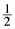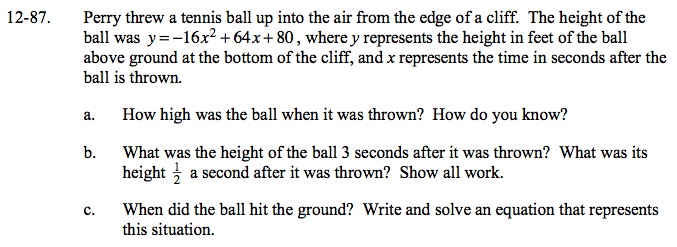### Home > GC > Chapter 12 > Lesson 12.2.4 > Problem12-87

12-87.
1. Perry threw a tennis ball up into the air from the edge of a cliff. The height of the ball was y = −16xs2 + 64x + 80 , where y represents the height in feet of the ball above ground at the bottom of the cliff, and x represents the time in seconds after the ball is thrown. Homework Help ✎

1. How high was the ball when it was thrown? How do you know?

2. What was the height of the ball 3 seconds after it was thrown? What was its heighta second after it was thrown? Show all work.

3. When did the ball hit the ground? Write and solve an equation that represents this situation.Substitute 0 in for x.

y = -16(0²) + 64(0) + 80 = 80 feet

Substitute 3 for x in the given equation.

y = -16(3²) + 64(3) + 80
y = -144 + 192 + 80
y = -144 + 272
y = 128 ft

y = -16(0.5²) + 64(0.5) + 80
y = -4 + 32 + 80
y = -4 + 112
y = 108 ft

If the ball hits the ground, what is its height above the ground?

-16x² + 64x + 80 = 0

−16(x² − 4x − 5)
−16(x − 5)(x + 1)
x = 5 seconds